#### 期刊菜单

Research on Grid-Connected Control Strategy of an AC and DC Power Router
DOI: 10.12677/SG.2022.122003, PDF, HTML, XML, 下载: 144  浏览: 254

Abstract: The application of power routers in the energy Internet increases the diversity of power supply, and also solves the problem of multi-directional power flow. In this paper, a small-capacity single-phase AC and DC power router is designed according to the actual needs of home users. The AC and DC systems are connected by bidirectional DC/AC converters to ensure reliable power supply to the load. Since the output of distributed power is easily fluctuated by the weather, this paper proposes an improved sliding average algorithm for the grid-connected control strategy of power routers, which can obtain the output command power of distributed power. By controlling the distributed power output to track the command power, a smooth grid-connected power can be obtained and fluctuations can be reduced. Finally, the correctness of the proposed grid-connected control method is verified by simulation.

1. 引言

2. 电能路由器设计原则及其拓扑结构

2.1. 电能路由器设计原则

1) 分布式电源和负载的即插即用

2) 交直流母线稳定

3) 孤网并网状态快速切换

4) 能量的多向流动

5) 能量管理优化保证功率平衡与功率平滑

2.2. 电能路由器拓扑结构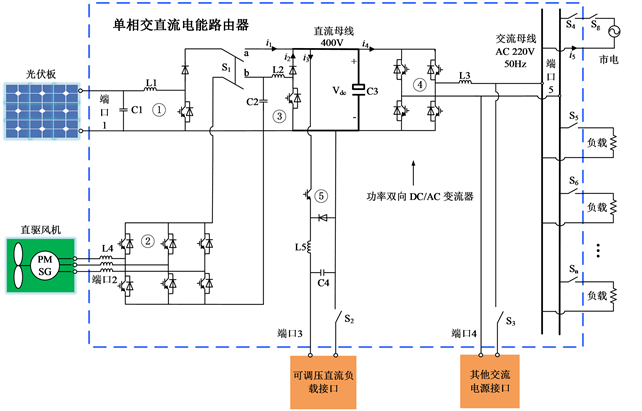Figure 1. The topology diagram of the single-phase AC and DC power router proposed in this paper

3. 电能路由器的并网控制方法

3.1. 并网目标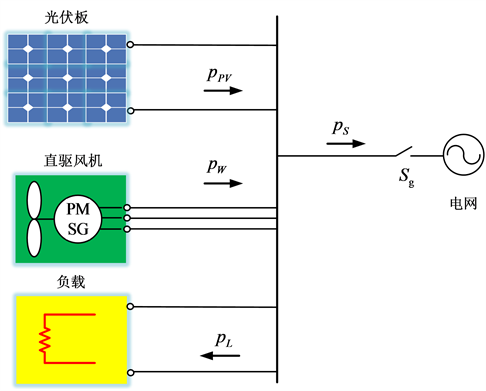Figure 2. System power flow in grid-connected state

${P}_{\text{S}}={P}_{\text{PV}}+{P}_{\text{W}}-{P}_{\text{L}}$ (1)

3.2. 滑动平均法

${f}_{k}={y}_{k}=\frac{1}{2n+1}{\sum }_{k=-n}^{n}{y}_{k+1},\text{\hspace{0.17em}}\text{\hspace{0.17em}}k=n+1,n+2,\cdots ,N-n$ (2)

3.3. 并网点功率平滑控制策略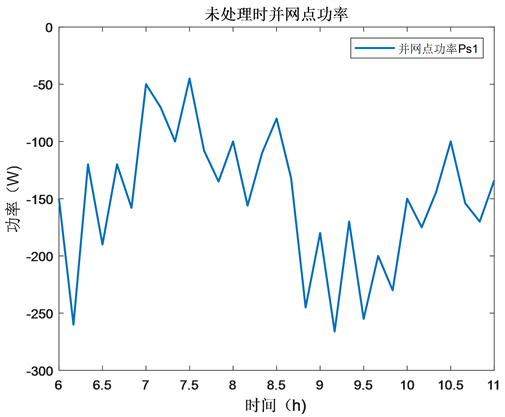Figure 3. Grid-connected power without smoothing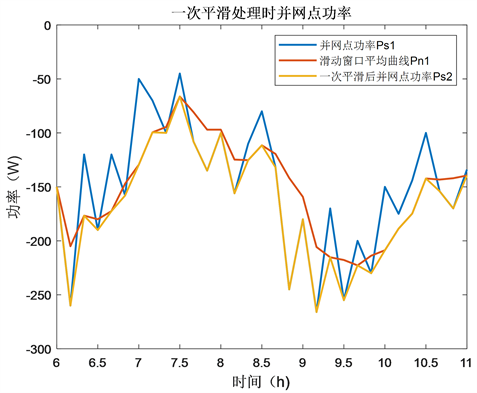Figure 4. Grid-connected power when processed by moving average algorithm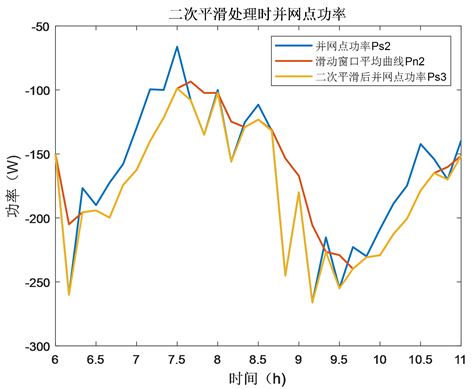Figure 5. Grid-connected power when processed by quadratic moving average algorithm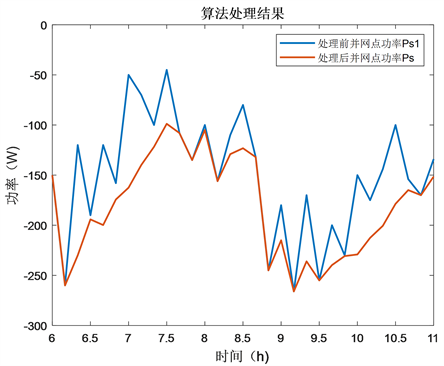Figure 6. Grid-connected power when processed by grid-connected control strategy methodTable 1. The fluctuation of the power of the grid connection point after each algorithm processing

4. 仿真验证

4.1. 仿真模型参数

SPWM调制载波频率：f = 5 kHz。

PI参数：电压环kp = 1，ki = 30；电流环kp = 0.01，ki = 2。

4.2. 基本功能验证

4.2.1. 并网预同步与功率双向流动Figure 7. Waveform diagram of inverter tracking grid phase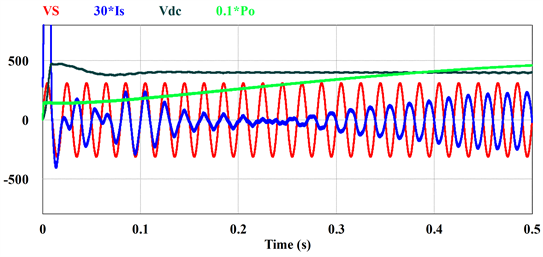Figure 8. Experimental waveforms for bidirectional power flow

4.2.2. 光伏单独并网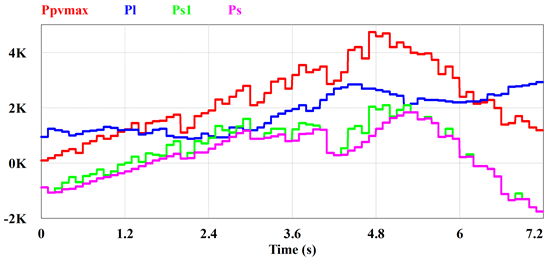Figure 9. Grid-connected simulation waveform when PV works alone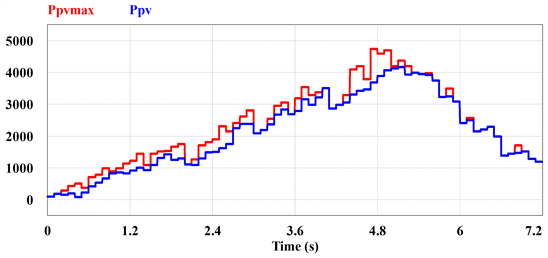Figure 10. The maximum power and actual output power of photovoltaics obtained by simulation

4.2.3. 风光联合出力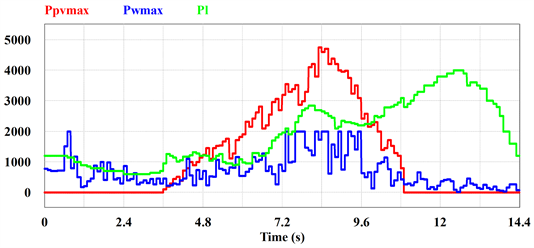Figure 11. The curve of the maximum power of photovoltaic and wind turbines and the load power in a day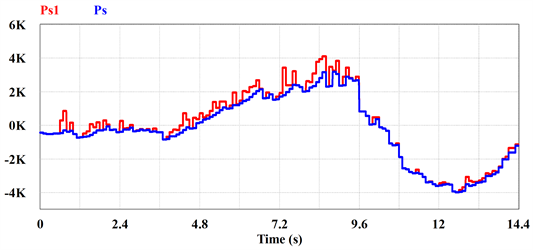Figure 12. Grid-connected power when wind power and photovoltaic work together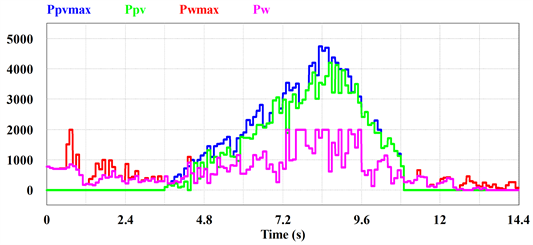Figure 13. The maximum power and actual output power of wind and photovoltaics obtained by simulation

5. 结论

  吴聪, 唐巍, 白牧可, 等. 基于能源路由器的用户侧能源互联网规划[J]. 电力系统自动化, 2017, 41(4): 20-28.  孟明, 陈世超, 赵树军, 等. 新能源微电网研究综述[J]. 现代电力, 2017, 34(1): 1-7.  郭慧, 汪飞, 张笠君, 罗建. 基于能量路由器的智能型分布式能源网络技术[J]. 中国电机工程学报, 2016, 36(12): 3314-3325.  宗升, 何湘宁, 吴建德, 李武华, 赵荣祥. 基于电力电子变换的电能路由器研究现状与发展[J]. 中国电机工程学报, 2015, 35(18): 4559-4570.  周孝信, 鲁宗相, 刘应梅, 等. 中国未来电网的发展模式和关键技术[J]. 中国电机工程学报, 2014, 34(29): 4999-5009.  查亚兵, 张涛, 黄卓, 等. 能源互联网关键技术分析[J]. 中国科学(信息科学), 2014, 44(6): 702-713.  徐梦超, 邰能灵, 黄文焘, 郑晓冬, 李国栋, 陈培育, 王旭东. 基于社区能源网的电能路由器设计[J]. 电力系统保护与控制, 2016, 44(23): 177-183.  廖志凌, 阮新波. 独立光伏发电系统能量管理控制策略[J]. 中国电机工程学报, 2009, 29(21): 46-52.  Dall’Anese, E., et al. (2014) Optimal Dispatch of Photovoltaic Inverters in Residential Distribution Systems. IEEE Transactions on Sustainable Energy, 5, 487-497. https://doi.org/10.1109/TSTE.2013.2292828  Bahrami, S., Parniani, M. and Vafaeimehr, A. (2012) A Modified Approach for Residential Load Scheduling Using Smart Meters. 2012 3rd IEEE PES Innovative Smart Grid Technologies Europe (ISGT Europe), Berlin, 14-17 October 2012, 1-8. https://doi.org/10.1109/ISGTEurope.2012.6465717  郭骞. 新能源电站中储能电池技术的对比与发展前景预测[J]. 太阳能, 2021(12): 5-10.  吴宇奇, 叶雨晴, 马啸, 王子璇, 李正天, 徐海波. 抑制电压波动与规避频率越限的孤岛微电网并网预同步方案[J]. 电力系统自动化, 2021, 45(14): 56-64.  裴益轩, 郭民. 滑动平均法的基本原理及应用[J]. 火炮发射与控制学报, 2001(1): 21-23.